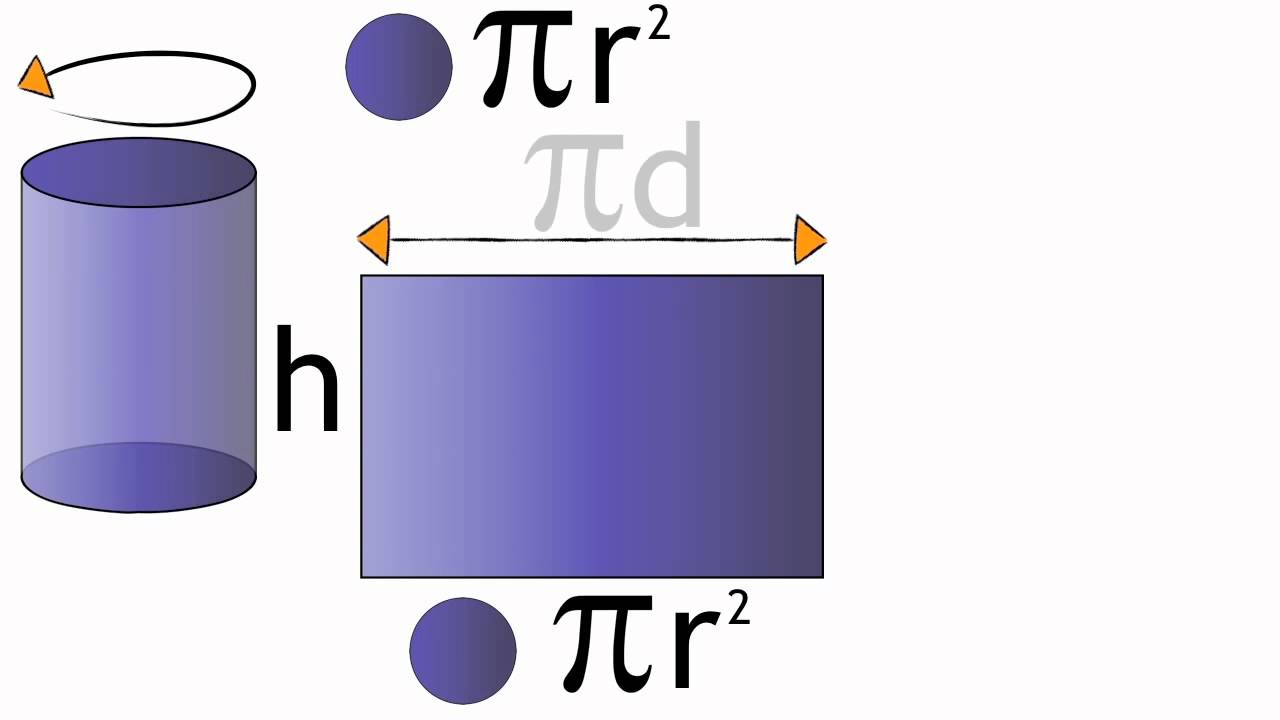A cylinder is a three-dimensional closed solid which has two identical bases connected by a curved surface. The bases are usually circular in shape and it should be congruent and parallel to each other. A cylinder is said to be right cylinder when the axis produces right angle to the base. If the axis does not produce the right angle to the base and one of the bases is displayed sideways, it is said to be an oblique cylinder. It is noted that the shapes with a curved surface have a surface area. To obtain the Surface area of the cylinder, it is necessary to find the area of both the bases and the area of the outer wall of a cylinder and then add both the areas.The steps involved in finding the area are :

• Calculate the base areas
• Calculate the area of the outer wall
• Factorise the terms

Since both the bases are circular in shape, find the area of a circle which is found from the radius ‘ r ‘  of a circle. We know that the area of a circle is defined as πr2. So, the combined area for both the top and bottom base is twice and the area is given as 2πr2.

When unrolling the cylinder, a rectangular side is obtained. In other words, the outer wall of a cylinder is a rectangle. The area of a rectangle is given as width times length. Here, the width and length refers to the height and the distance around the end circles. This denotes the circumference of a circle and therefore the area of the outer rectangular wall is 2πr x h. When combining both areas, it becomes

Surface Area =  2πr2 + 2πr x h

To simplify this, use the factorisation method. Take 2πr as common, the formula for surface area is written as

Surface Area = 2πr ( r + h ) Square units.

Similarly, it is easy to derive the surface area of three-dimensional solids by calculating the area of two-dimensional shapes. Because the basis of 3D shapes is 2D shapes. By rotating 2D shapes, 3D shapes are formed.

Subscribe to BYJU’S YouTube channel for more such information on 2D and 3D shapes and also watch engaging videos to learn with ease.